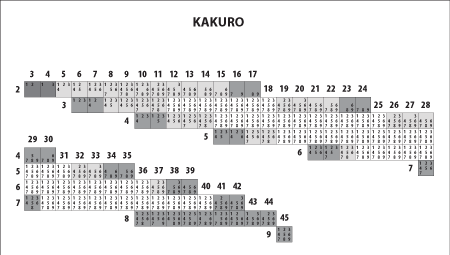﻿ Kakuro Combinations | Christoph Rüegg

Enough Sudoku for a while? Try Kakuro!

I've created a small helper table lately, listing what numbers are possible in what condition (dependent on the required sum and the number of fields). You may download it as a PDF:I could have evaluated the allowed numbers by hand, but decided to write a small console code snippet in C# instead. It's neither optimized nor well designed, but it works... note to myself: use a functional language next time

Call the method Kakuro(s,f) with the required sum in s and the number of fields in f, and it returns all possible combinations (only the ascending permutation for each) and the union set of numbers appearing in at least one combination (that's the numbers in the helper table above)

The recursive backtracking method (with inline console output for simplicity):

  1: 2: 3: 4: 5: 6: 7: 8: 9: 10: 11: 12: 13: 14: 15: 16: 17: 18: 19: 20: 21: 22:  static BitArray AppendSummand(int summandsLeft, int targetSum, BitArray used, int sum, int nextSummand) { if(summandsLeft == 0) { if(targetSum == sum) { // found valid solution PrintUsed(used, false); return used; } else // invalid solution A return new BitArray(9); } if(nextSummand >= 10) // invalid solution B return new BitArray(9); BitArray usedWith = new BitArray(used); usedWith[nextSummand-1] = true; // try with this number BitArray resultWithout = AppendSummand(summandsLeft, targetSum, used, sum, nextSummand+1); BitArray resultWith = AppendSummand(summandsLeft-1, targetSum, usedWith, sum+nextSummand, nextSummand+1); // combine results for the summary return resultWithout.Or(resultWith); } 

And the method to run it:

  1: 2: 3: 4: 5: 6: 7: 8: 9: 10:  static void Kakuro(int sum, int fields) { Console.WriteLine("=================================="); Console.WriteLine("** Sum {0} in {1} cells",sum,fields); BitArray used = new BitArray(9); BitArray res = AppendSummand(fields,sum,used,0,1); Console.Write("** Superset: "); PrintUsed(res, true); Console.WriteLine(); } 

Finally the print method used to print the numbers to the console:

  1: 2: 3: 4: 5: 6: 7: 8: 9: 10: 11: 12: 13: 14: 15: 16:  static void PrintUsed(BitArray used, bool all) { Console.ForegroundColor = ConsoleColor.Green; if(all) for(int i=0;i